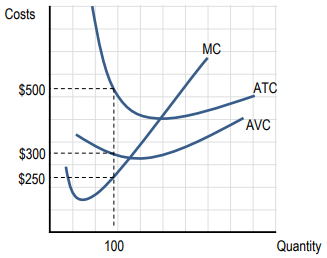Clutch Prep is now a part of Pearson
Ch. 10 - The Costs of ProductionWorksheetSee all chapters

# Graphing Costs

See all sections
Sections
Revenue, Cost, and Profit
The Production Function and Diminishing Returns
Marginal Cost
The Relationship Between Average Cost and Marginal Cost
Graphing Costs
Average Total Cost: Short Run and Long Run
Isoquant Lines
Isocost Lines
Cost-Minimizing Combination of Labor and Capital
###### One of the most important graphs in microeconomics!

Concept #1: Graphing Cost Curves

Practice: If average total cost is $50, quantity produced is 10 and total fixed cost$100, what is the total variable cost for the output of 10?

Practice: Based on the graph below, at a quantity of 100, AFC is equal to:Practice: When a firm is producing zero output, total cost equals: